Next: Rational B-splines Up: Some Mathematics for Advanced Previous: Bezier curves

Subsections

# B-splines

B-splines are a more general type of curve than Bezier curves. In a B-spline each control point is associated with a basis function.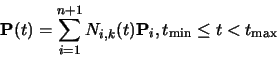(87)

There are n + 1 control points,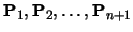. The Ni,k basis functions are of order k(degree k-1). k must be at least 2 (linear), and can be no more than n+1 (the number of control points). The important point here is that the order of the curve (linear, quadratic, cubic,...) is therefore not dependent on the number of control points (which it is for Bezier curves, where k must always equal n+1).

Equation 87 defines a piecewise continuous function. A knot vector,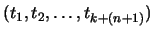, must be specified. This determines the values of t at which the pieces of curve join, like knots joining bits of string. It is necessary that: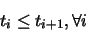(88)

The Ni,k depend only on the value of k and the values in the knot vector. N is defined recursively as:

 Ni,1(t) =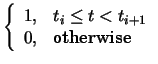Ni,k(t) =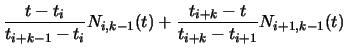(89)

This is essentially a modified version of the idea of taking linear interpolations of linear interpolations of linear interpolations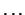n

At this point it would be instructive for you to work out N1,1, N2,1, N3,1, N1,2, N2,2, N1,3 for the knot vector (0,2,3,6). It helps if you draw the graphs for these functions.

There are several things that you should note about these equations. Each Ni,k(t) depends only on the k+1 knot values from ti to ti+k. Ni,k(t)=0 for t < ti or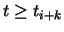so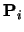only influences the curve for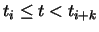. Formally,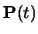is a polynomial of order k (degree k-1) on each interval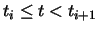. Across the knotsis Ck-2-continuous.is, of course, continuous in all its derivatives between the knots.is validly defined for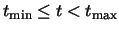where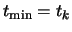and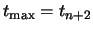. Even more properties of B-splines are described in Rogers and Adams pp. 306-7.

## The knot vector

The above explanation shows that the knot vector is very important. The knot vector can, by its definition, be any sequence of numbers provided that each one is greater than or equal to the preceding one. Some types of knot vector are more useful than others. Knot vectors are generally placed into one of three categories: uniform, open uniform, and non-uniform.

Uniform.
These are knot vectors for which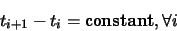(90)

For example: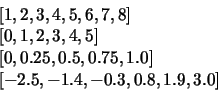Open Uniform.
These are uniform knot vectors which have kequal knot values at each end: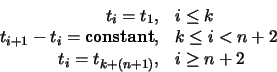(91)

For example: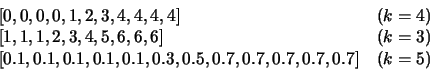Non-uniform.
This is the general case, the only constraint being the standard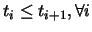(Equations 88). For example: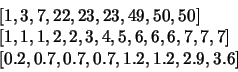The shapes of the Ni,k basis functions are determined entirely by the relative spacing between the knots. Scaling (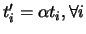) or translating (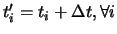) the knot vector has no effect on the shapes of the Ni,k.

The above gives a description of the various types of knot vector but it doesn't really give you any insight into how the knot vector determines the shape of the curve. The following subsections look at the different types of knot vector in more detail. However, the best way to get to feel for these is to derive and draw the basis functions yourself.

### Uniform knot vector

For simplicity, let ti = i (this is allowable given that the scaling or translating the knot vector has no effect on the shapes of the Ni,k). The knot vector thus becomes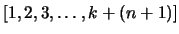and Equation 89 simplifies to:

 Ni,1(t) =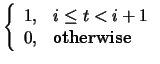Ni,k(t) =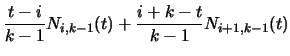(92)

You should be easily able to graph the first few of these for yourself. The principle thing to note about the uniform basis functions is that, for a given order k, the basis functions are simply shifted versions of one another.

### Things you can change about a uniform B-spline

With a uniform B-spline, you obviously cannot change the basis functions (they are fixed because all the knots are equispaced). However you can alter the shape of the curve by modifying a number of things:
Moving control points.
Moving the control points obviously changes the shape of the curve.
Multiple control points.
Sticking two adjacent control points on top of one another causes the curve to pass closer to that point. Stick enough adjacent control points on top of one another and you can make the curve pass through that point.
Order.
Increasing the order k increases the continuity of the curve at the knots, increases the smoothness of the curve, and tends to move the curve farther from its defining polygon.
Joining the ends.
You can join the ends of the curve to make a closed loop. Say you have M points,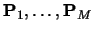. You want a closed B-spline defined by these points. For a given order, k, you will need M+(k-1) control points (repeating the first k-1 points):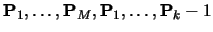. Your knot vector will thus have M+2k-1 uniformly spaced knots.

### Open uniform knot vector

The previous section intimated that uniform B-splines can be used to describe closed curves: all you have to do is join the ends as described above. If you do not want a closed curve, and you use a uniform knot vector, you find that you need to specify control points at each end of the curve which the curve doesn't go near.

If you wish your B-spline to start and end at your first and last control points then you need an open uniform knot vector. The only difference between this and the uniform knot vector being that the open uniform version has k equal knots at each end.

An order k open uniform B-spline with n+1=k points is the Bezier curve of order k. It would be a useful exercise for you to prove this for k=3. For ease of calculation take the knot vector to be [0,0,0,1,1,1].

### The difference between uniform and open uniform

It may help, at this stage, to compare a particular uniform and an equivalent open uniform knot vector. Unfortunately the translation from LaTeX to HTML couldn't cope with this section of the notes, so it has had to be deleted. It appears in the paper version.

### Non-uniform knot vector

Any B-spline whose knot vector is neither uniform nor open uniform is non-uniform. Non-uniform knot vectors allow any spacing of the knots, including multiple knots (adjacent knots with the same value). We need to know how this non-uniform spacing affects the basis functions in order to understand where non-uniform knot vectors could be useful. It transpires that there are only three cases of any interest: (1) multiple knots (adjacent knots equal); (2) adjacent knots more closely spaced than the next knot in the vector; and (3) adjacent knots less closely spaced than the next knot in the vector. Obviously, case (3) is simply case (2) turned the other way round.

Multiple knots.
A multiple knot reduces the degree of continuity at that knot value. Across a normal knot the continuity is Ck-2. Each extra knot with the same value reduces continuity at that value by one. This is the only way to reduce the continuity of the curve at the knot values. If there are k-1 (or more) equal knots then you get a discontinuity in the curve.
Close knots.
As two knots' values get closer together, relative to the spacing of the other knots, the curve moves closer to the related control point.
Distant knots.
As two knots' values get further apart, relative to the spacing of the other knots, the curve moves further away from the related control point.

### Use of non-uniform knot vectors

Standard procedure is to use uniform or open uniform B-splines unless there is a very good reason not to do so. Moving two knots closer together tends to move the curve only slightly and so there is usually little point in doing it. This leads to the conclusion that the main use of non-uniform B-splines is to allow for multiple knots, which adjust the continuity of the curve at the knot values.

However, non-uniform B-splines are the general form of the B-spline because they incorporate open uniform and uniform B-splines as special cases. Thus we will talk about non-uniform B-splines when we mean the general case, incorporating both uniform and open uniform.

### What can you do to control the shape of a B-spline?

• Move the control points.
• Add or remove control points.
• Use multiple control points.
• Change the order, k.
• Change the type of knot vector.
• Change the relative spacing of the knots.
• Use multiple knot values in the knot vector.

### What should the defaults be?

If there are no pressing reasons for doing otherwise, your B-spline should be defined as follows:
• k=4 (cubic);
• no multiple control points;
• uniform (for a closed curve) or open uniform (for an open curve) knot vector.

## B-spline patches

We generalise from B-spline curves to B-spline surfaces in the same way as we did for Bezier patches. Take a tensor product of two versions of Equation 87.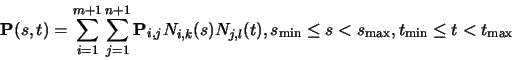(93)

where it is usual for the patch to have the same order (i.e. k=l) in both directions.Next: Rational B-splines Up: Some Mathematics for Advanced Previous: Bezier curves